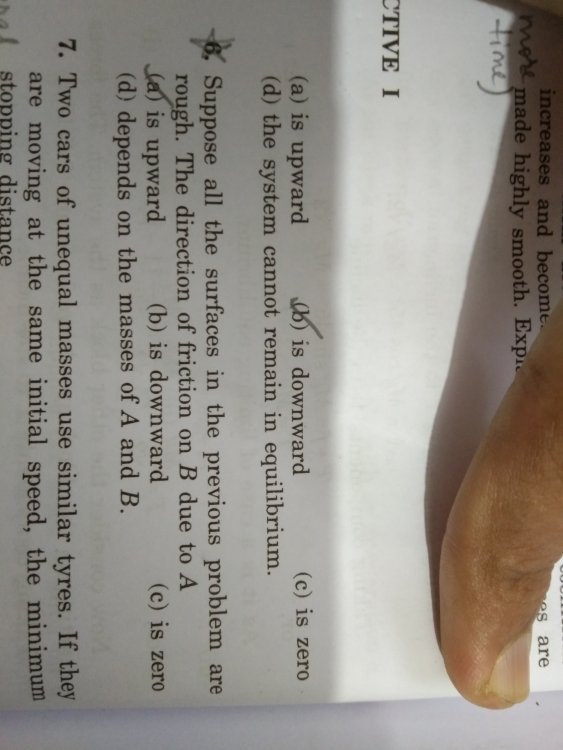Friction Question 2

Recommended Posts

I am having trouble understanding this question. The questions asks the direction of friction. The correct answer is the is that the system cannot remain in equilibrium.

One more question is that if the friction coefficient between A and the wall had not been zero what would have been the direction of friction due to A on B then?

Share on other sites

If "the surfaces of A and B are rough" then there is enough friction between them that they do not move relative to each other. Since A and B are in equilibrium the force between A and B must be F.

Share on other sites

• Phi for All changed the title to Friction Question 2
2 hours ago, Arnav said:

I am having trouble understanding this question. The questions asks the direction of friction. The correct answer is the is that the system cannot remain in equilibrium.

One more question is that if the friction coefficient between A and the wall had not been zero what would have been the direction of friction due to A on B then?

This question is incomplete.

Share on other sites

@studiot do you mean that the magnitude of coefficient of friction, the weight of the blocks is not given? or is the question incorrect?

Share on other sites

3 hours ago, Arnav said:

@studiot do you mean that the magnitude of coefficient of friction, the weight of the blocks is not given? or is the question incorrect?

Neither.

You haven't posted the whole question.

"The friction on B due to A in equilibrium"

is an incomplete sentence that may or may not contain the actual question.

Further the blocks must be sliding down the wall if there is no friction between the wall and the blocks.
So how is F maintained ?

Share on other sites

here is the rest of the 5th question, the correct answer is given as the 4th optioni think by equilibrium the author means to ask whether the force force F on itself,alone, will it be able to keep the blocks in equilibrium?

Share on other sites

If you think about my free body diagrams in your other friction questions and draw similar ones here you can answer both question 5

Are the blocks in equilibrium ?

and

question 6 which follows it.

Since the wall is smooth, both blocks must be falling freely under gravity.

Do objects of unequal weight fall at different speeds ?

Create an account

Register a new account